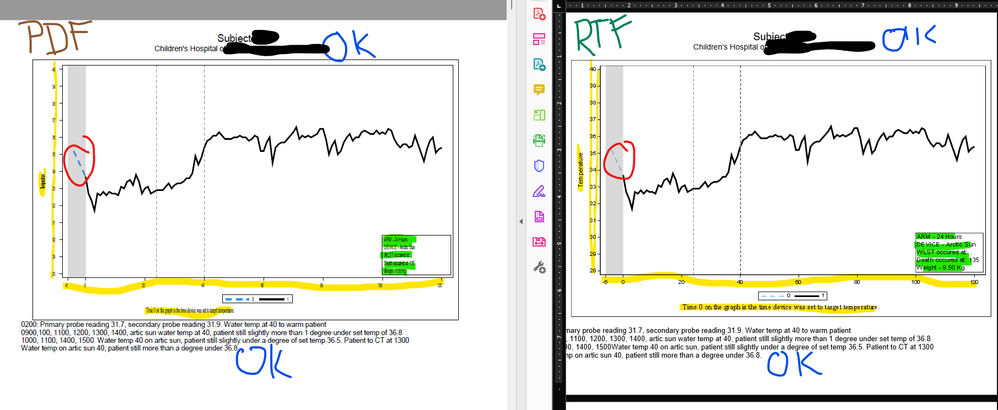## ODS Changes to PDF and RTF

After our IT group updated SAS on our local machines and we now run the latest version of EG, programs that a group of us have that used to output to PDF were doing some unexpected things. Text inside the SG procedure such as axis labels and values were distorted. Quickly we switch to RTF since the output was more consistent.

However, now we are running into problems there as well. First the singular problem I have with the RTF on the right side of the picture. I have an attribute map dataset that is conditionally coloring segments of the series line (Blue and Black). If i specify a pattern in the attribute map for then LineThickness= does not yield any results. When I change RTF to PDF I get the results for the line that I want.

But this leads me back to my original problem of all the things that I have highlighted that are wrong. Axis values and labels are tiny and the wrong font, added space in the inset of the graph, etc.

The only thing that remains unchanged is the ODS RTF/PDF text="". So Im sure it is an interaction happening with the SGPlot procedure and ODS.

Attached is the SGPlot code and the different outputs. Can someone tell me why this is happening?

``````data discreteMap;
length id \$3 value \$3 LineColor \$50   linepattern \$ 3;
input id \$ value \$ linecolor \$  linepattern \$;
datalines;
X 0 dodgerblue  20
X 1 black  1
;
run;

%macro Subject_graphs;

ods escapechar="~";
proc sort data=work.overall_dataset;
by zsubjectid &Time.;
run;
options orientation=landscape  spool;
ods graphics on / imagemap height=5.5in width=9in   ;
ODS pdf File="C:\Users\clevenge\Desktop\Grey\SAS_Batch\PEDSICECAP\Weekly_Temps\Week_of_&today.\Test Franks Subject Graphs &asofdt..pdf" STYLE=rtf  ;
%let N_sub=%sysfunc(countw(&subj_list.));
%do q=1 %to &N_sub;
%let subj=%scan(&subj_list,&q);
%if &subj. gt 1077 %then %do;
ods pdf startpage=never;
ods pdf text="~S={Leftmargin=1in Rightmargin=1in outputwidth=100% font=(Arial,16pt) just=c foreground=black} Subject &Subj. ";
ods pdf text="~S={Leftmargin=1in Rightmargin=1in outputwidth=100% font=(Arial,12pt) just=c foreground=black} %str(&&&S_&subj.) ";
title;

proc sgplot data=work.Overall_Solid_line  dattrmap=discreteMap  ;
where  zSubjectID= &subj ;
series x=&Time. y=sub_avg / name="Line" group=line_grp attrid=X lineattrs=(thickness=3px) ;
inset "ARM - &&&T_&subj. " "DEVICE - &&&D_&subj. " "&&&W_&Subj. " "&&&N_&subj. " "Weight - &&&k_&subj. Kg" /  BORDER position=bottomright  textattrs=(family="Arial" Size=10 weight=normal)  ;
xaxis values=(-6 0 20 40 60 80 100 120) offsetmin=.015 offsetmax=.03 valueattrs=(family="Arial" size=8pt) display=(NOLABEL) ;
yaxis values=(28 to 40) offsetmax=.02 offsetmin=.02 valueattrs=(family="Arial" size=8pt) labelattrs=(family="Arial" size=10pt);
band y=sub_avg lower=-6 upper=0 / fill fillattrs=(color=gray ) transparency=.7 name="Band";
keylegend "Line" / valueattrs=(family="Arial" size=8pt) ;
refline sub_cool / axis=x lineattrs=(pattern=2);
refline sub_warm / axis=x lineattrs=(pattern=2);
footnote "Time 0 on the graph is the time device was set to target temperature. " j=center font="Arial" h=10pt ;
;
run;
footnote;
%if %symexist(Excersion_list_&subj._1) %then %do;
ods pdf text="~S={Leftmargin=.5in Rightmargin=.5in outputwidth=100% font=(Arial,11pt) just=l foreground=black}&&&Excersion_list_&subj._1 ";
%end;
%if %symexist(Excersion_list_&subj._2)  %then %do;
ods pdf text="~S={Leftmargin=.5in Rightmargin=.5in outputwidth=100% font=(Arial,11pt) just=l foreground=black}&&&Excersion_list_&subj._2 ";
%end;
%if %symexist(Excersion_list_&subj._3)  %then %do;
ods pdf text="~S={Leftmargin=.5in Rightmargin=.5in outputwidth=100% font=(Arial,11pt) just=l foreground=black}&&&Excersion_list_&subj._3 ";
%end;
%if %symexist(Excersion_list_&subj._4)  %then %do;
ods pdf text="~S={Leftmargin=.5in Rightmargin=.5in outputwidth=100% font=(Arial,11pt) just=l foreground=black}&&&Excersion_list_&subj._4 ";
%end;

ods pdf startpage=now;
%end;
%end;
ods _all_ close;
%mend;
%Subject_graphs;``````2 REPLIES 2

## Re: ODS Changes to PDF and RTF

Generic comment on using PIXELS as a unit of size: It changes. There is no standard definition of pixel.

I would suggest changing units to IN , MM, CM or PT (adjust values as needed) for portable sizes of graphing elements.

I'm not sure if just upgrading SAS would change the default value of pixel size in your installation but I don't have your installation.

Wrong font I'm not sure about. I had that happen at an upgrade because a custom ODS style I used had the parent style definition change so that the instructions for the custom style didn't have the base values the same and custom style needed to be redefined to align with the new SAS supplied parent style.

## Re: ODS Changes to PDF and RTF

goptions device=SASPRTC;

ods _all_ close;

Discussion stats
• 2 replies
• 236 views
• 1 like
• 3 in conversation